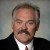# How Do I Determine Defects Per Million (DPM) for Sigma Values Greater Than 6 (3.4 DPM)?

ByDr. Mikel Harry

Once is a great while, there is a need to estimate or otherwise approximate the tail probability of an extreme standard normal deviate – such as Z = 7, Z = 10, or even higher.  For example, certain types of reliability problems rely on such assessments. But as many already know, most published tables of “area under the normal curve” terminate at about 4s. To our dismay, electronic spreadsheets “blow up” at around 6s. So what is one to do?

Handpicked Content:   Use Earned Value Management for Process Improvement

In an effort to satisfy this need, it is possible to devise a polynomial equation. However, most equations of this variety do tend to suffer from an inherent, progressive, and expanding rate of error. While there are other methods of calculation that are somewhat more precise (in a relative sense), those methods are also far more complex, difficult to work with, and are still subject to a certain amount of theoretical bias (error). After all, the ability to compute a fully “precise” tail probability for some extreme Z value can be somewhat of an esoteric and lofty exercise.

To convert a one-sided standard normal deviate ( Z ) to its corresponding tail area ( P ), we must compute the quantity:

P = [ ( 1 + C1*Z + C2*Z^2 + … + C6*Z^6)^-16 ]  /  2

where,

C1 = 0.0498673470
C2 = 0.0211410061
C3 = 0.0032776263
C4 = 0.0000380036
C5 = 0.0000488906
C6 = 0.0000053830.

To convert a one-sided tail area ( P ) to its corresponding standard normal deviate ( Z ), we must compute the quantity:

Z = L – [ ( C1 + C2*L + C3*L^2 ) / ( 1 + C4*L + C5*L^2 + C6*L^3) ]

where, L = sqrt[ ln ( 1 / P^2 ) ] and

C1 = 2.515517
C2 = 0.802853
C3 = 0.010328
C4 = 1.432788
C5 = 0.189269
C6 = 0.000308.

With the given equations and coefficients, a table of area under the normal curve can be easily configured and constructed. In fact, it can be built in such a manner so as to meaningfully extend well beyond the classic constraint of Z = 4.0.

But again, we are dutifully reminded that the error surrounding an estimate of P will disproportionately increase as Z progressively increases in magnitude. Of course, the inverse of this is also true – the relative error in our estimation of Z will disproportionately increase as the tabulated value of P is progressively diminished. Naturally, the pragmatic and theoretical consequences of this effect must always be rationally adjudicated prior to application.